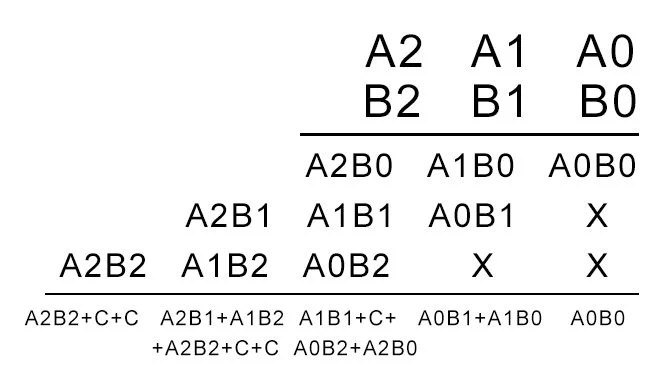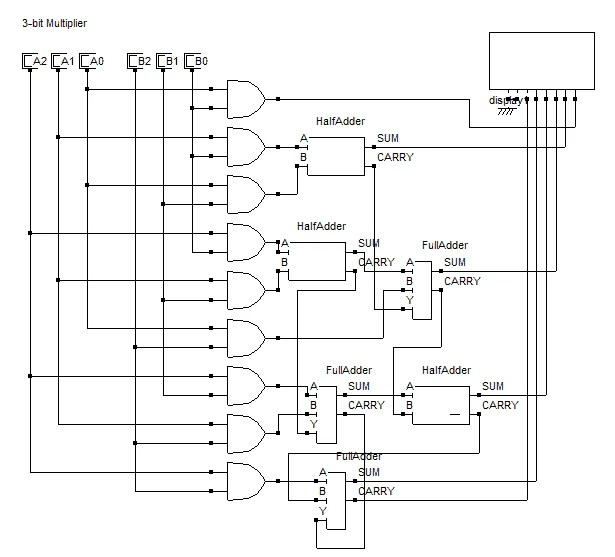View Course Path

# Multiplier – Designing of 2-bit and 3-bit binary multiplier circuits

A multiplier is a combinational logic circuit that we use to multiply binary digits. Just like the adder and the subtractor, a multiplier is an arithmetic combinational logic circuit. It is also known as a binary multiplier or a digital multiplier.

Contents

## Where is the use of a multiplier?

We use a multiplier in several digital signal processing applications. We use it to design calculators, mobiles, processors, and digital image processors.

## How does binary multiplication work and how to design a 2-bit multiplier?

Binary multiplication works just like normal multiplication. There are four main rules that are quite simple to understand:

0  x  0  =  0

0  x  1  =  0

1  x  0  =  0

1  x  1  =  1

Suppose you have two binary digits A1A0 and B1B0, here’s how that multiplication would take placeIn the above calculation, A1A0 is the multiplicand. B1B0 is the multiplier. The first product obtained from multiplying B0 with the multiplicand is called as partial product 1. And the second product obtained from multiplying B1 with the multiplicand is known as the partial product 2.

As the number of bits increases, we keep shifting each successive partial product to the left by 1 bit. In the end, we add the digits while keeping in mind the carry that might generate.

Based on the above equation, we can see that we need four AND gates and two half adders to design the combinational circuit for the multiplier. The AND gates will perform the multiplication, and the half adders will add the partial product terms. Hence the circuit obtained is as follows.If you would like to brush up your knowledge of digital logic gates, we’ve got you covered fam. Just click on the link.

## How to design a 3-bit multiplier?

Consider two general 3-bit binary numbers A2A1A0 and B2B1B0. Multiplying the two numbers with each other using standard binary arithmetic rules, we get the following equation.Adding A2B0 and A1B1 will give rise to one carry, adding the sum obtained from that, and the carry obtained from adding A1B0 and A0B1 to A0B2 will give rise to another carry. Thus, two carries are generated and are carried over to the addition between A2B1 and A1B2, where two more carries are created similarly.

Hence the resulting circuit will contain nine AND gates, three half adders, and three full adders. The resultant circuit is given below. We explain half and full adders in detail here.Note: We will use all of the equations above when we code multipliers in our VHDL and Verilog course.

## 6 thoughts on “Multiplier – Designing of 2-bit and 3-bit binary multiplier circuits”

1.Ahmed Mansour says:

how can i build the 3-bit multiplier circuit if the Left Most Bit is 1 for -ve and 0 for +ve???
example: a(1 0 1)=-1 * b(0 1 1)=3

2.DUC says:

I need help with the 3-bit multiplier vhdl code using structural,plz help me

3.Sengul Celik says:

Sir Would you help me to draw gate for with HA and FA other to perform 3x where x is greater and equal to zero and less then 8 with 3 inputs and 5 outputs. Thank you in advance.
My name is Sue from Macquarie Uni Sydney

1.Umair Hussaini says:

I think you missed the keyword in your comment. What functionality are you trying to achieve? What logic are you trying to implement?

4.Mahreen Asama says:

Sir kindly draw the truth table of this circuit

1.Umair Hussaini says:

You can easily get the truth tables for the 2-bit and 3-bit multipliers by putting all possible values of the inputs in the equations we generated above. However, there is truly no need to. Because these truth tables will be quite extensive and convoluted to solve.

This site uses Akismet to reduce spam. Learn how your comment data is processed.